This blog is managed by Song Hock Chye, author of Improve Your Thinking Skills in Maths (P1-P3 series), which is published and distributed by EPH.

## Wednesday, March 25, 2009

### Tao Nan School P5 SA2 2006 Math Q48

At an exhibition, 30% of those present were boys. 5/14 of the remainder were girls and the rest were adults. There were 45 more adults than boys. How many more children than adults were there at the exhibition?

Solution

Boys ----- 30%
Girls ----- 5/14 x 70% = 25%
Adults ----- 100% - 30% - 25% = 45%

Adults more than boys ---- 45
45% - 30% ----- 45
15% ----- 45 (more adults than boys)
1% ----- 45 divided by 15 = 3

30% (boys) + 25% (girls) – 45% (adults) = 10%
10% ----- 10 x 3 = 30

### Tao Nan School P5 SA2 2006 Math Q47

Ricci saved \$200 from her salary and spent the rest. She spent 1/9 of the expenditure on a blouse, \$40 on a scarf and the rest on books. The amount spent on the scarf was \$20 less than that spent on the blouse. What was her salary?

Solution1/9 of expenditure (blouse) ----- \$20 + \$40 (scarf) = \$60

9/9 of expenditure ----- 9 x \$60 = \$540

Salary ----- \$540 + \$200 (saved) = \$740

### Tao Nan School P5 SA2 2006 Math Q46

Cherie had 3 times as much money as Jolene. After Cherie spent \$40 and Jolene spent \$8, Jolene had 3 times as much money as Cherie. How much money did Cherie have at first?

Solution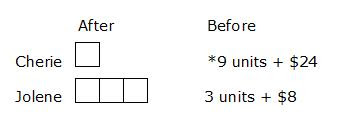Jolene (before) ----- 3 units + \$8
*Cherie (before) had 3 times as much as Jolene -----
(3 x 3 units) + (3 x \$8) = 9 units + \$24

Cherie (before) – Cherie (after) ----- \$40 (spent)
(9 units + \$24) – (1 unit) ----- \$40
8 units + \$24 ------ \$40
8 units ----- \$40 - \$24 = \$16
1 unit ----- \$16 divided by 8 = \$2

Cherie (at first)
9 units + \$24
= (9 x \$2) + \$24
= \$18 + \$24
= \$42

## Saturday, March 21, 2009

### Tao Nan School P5 SA2 2006 Math Q45

A box containing 58 iron balls weighed 2145g . when 46 of the iron balls were removed, the mass of the box and the remaining iron balls weighed 489g. What was the mass of the box?

Solution

Number of iron balls left when 46 were removed
58 – 46 = 12 (iron balls)

Box + 58 iron balls ----- 2145 g
Box + 12 iron balls ----- 489 g

(58 – 12) iron balls ----- 2145 g – 489 g
46 iron balls ----- 1656 g
1 iron ball ----- 1656 g divided by 46 = 36 g

Box + 58 iron balls ----- 2145 g
Box + (58 x 36 g) ----- 2145 g
Box + 2088 g ----- 2145 g
Box ----- 2145 g – 2088 g = 57 g

Answer: The box was 57 g.

## Wednesday, March 18, 2009

### Tao Nan School P5 SA2 2006 Math Q44

ABDE is a parallelogram and BCD is an isosceles triangle. Calculate
a) Angle DBC
b) Angle EAB
The figure below is not drawn to scale.Solution

a) Angle DBC ----- (180 – 42 – 42) degrees = 96 degrees (Answer)

b) Angle EAB = Angle EDB -----
(96 + 42) degrees = 138 degrees (Answer)

## Tuesday, March 17, 2009

### Tao Nan School P5 SA2 2006 Math Q43

A container measuring 17cm by 8cm by 10cm was half filled with water. When 8 cubes were put in, the water level became 8cm. Find the volume of each cube.

Solution

Height increase -----
8 cm (new height) – 5 cm (half tank) = 3 cm

Volume of 8 cubes -----
17cm x 8cm x 3cm
= 408 cubic cm

1 cube ----- 408 cubic cm divided by 8
= 51 cubic cm

### Tao Nan School P5 SA2 2006 Math Q42

Alex has some red pens and blue pens in the ratio of 7:3. He has 20 more red pens than blue pens. What is the new ratio of the number of red pens to the number of blue pens after he has given away 5 blue pens?

Solution

Red : Blue
7 : 3
Difference between blue and red -----
4 units ----- 20
1 unit ----- 5

Number of pens
Red ----- 7 x 5 = 35
Blue ----- 3 x 5 = 15

After 5 blue pens given away
Red ----- 35
Blue ----- 15 – 5 = 10

New ratio
Red : Blue
35 : 10
7 : 2

Answer: The new ratio of red pens to blue pens is 7:2

### Tao Nan School P5 SA2 2006 Math Q41

Faridah, Iman and Erin shared some money in the ratio of 8:5:3. Iman and Erin had a total of \$48. How much more money did Faridah have than Iman?

Solution

Faridah : Iman : Erin
8 : 5 : 3

Iman and Erin -----
8 units ----- \$48
1 unit ----- \$48 divided by 8 = \$6

Faridah more than Iman -----
3 units ----- 3 x \$6 = \$18

### Tao Nan School P5 SA2 2006 Math Q40

Joe had \$68 more than Mark. Bobbi had thrice as much as Mark. On the average, each person had \$56. How much money did Joe have?

Solution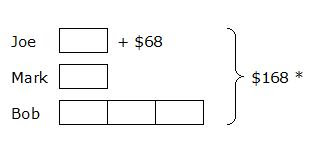* Average per person is \$56. Total amount is -----
3 x \$56 = \$168

5 units ----- \$168 - \$68 = \$100
1 unit ----- \$100 divided by 5 = \$20

(Joe) 1 unit + \$68 ----- \$20 + \$68 = \$88

### Tao Nan School P5 SA2 2006 Math Q39

An orange cost ½ as much as a watermelon. Jiamin paid \$21 for 12 watermelons and 6 oranges. How much did she pay for the 6 oranges?

Solution

Orange ----- 1 unit
Water Melon ----- 2 units

12 water melons + 6 oranges ----- \$21
(12 x 2 units) + 6 units ----- \$21
24 units + 6 units ----- \$21
30 units ----- \$21
1 unit ------ \$21 divided by 30 = \$0.70

(6 oranges) 6 units ----- 6 x \$0.70 = \$4.20

### Tao Nan School P5 SA2 2006 Math Q38

A shopkeeper bought 280 apples. 28 of them were rotten. He then sold 63 of the good ones to Seng and the rest to Ming. What fraction of the good apples did Ming buy? (Give your answer in the simplest form)

Solution

280 (apples) – 28 (rotten) = 252 (good apples)

252 – 63 = 189 (apples sold to Ming)

Fraction Ming bought ---- 189/252 = ¾

Answer: Ming bought ¾ of the apples.

## Wednesday, March 11, 2009

### Hormat Senjata in 2 moves

Our son completed his BMT today and what a difference NSF is today compared to what it was years ago.

For those who have done NS in the early days when the AR-15 (assault weapon) was in use, you’ll be amazed at how quick the hormat senjata can now be done - in just 2 moves – all because the clumsy AR-15 has been replaced by a shorter weapon.

Watch this video how clean and neat the hormat senjata is done – as compared to the old way.

Take note (picture below) that even in senang diri position (note the feet apart), the weapon is still slung over the shoulder – unlike the AR-15 that has to be put on the ground. This means that the soldiers can now hormat senjata from sedia position, and not from sedia, then rusuk senjata, then hormat senjata.

How things have changed.## Monday, March 09, 2009

### Tao Nan School P5 SA2 2006 Math Q37

If Elicia buys 2 kg of squids, she will have \$28.90 left. If she buys 5 kg of squids, she will have \$10.60 left. How much money does Elicia have?

Solution3 units ----- \$28.90 - \$10.60 = \$18.30
1 unit ----- \$18.30 divided by 3 = \$6.10

Elicia has
5 units + \$10.60
= 5 x \$6.10 + \$10.60
= \$41.10

### Tao Nan School P5 SA2 2006 Math Q36

Mary collected 75 sticks and Rose collected 43. After giving 22 sticks to their younger sister, they put the remainder equally into 4 boxes. How many sticks were there in each box?

Solution

Total collected ----- 75 + 43 = 118
Gave to sister ----- 118 – 22 = 96
96 (sticks) divided 4 (boxes) = 24

Answer: There were 24 sticks in each box.

## Tuesday, March 03, 2009

### Calculating the Area of a Triangle

Area of a triangle = ½ x base x height

A very common mistake students make is extending the base outside the triangle.
Example -
Question – Find the area of the shaded triangle.Common Mistake (Wrong solution)

A common mistake students make in the above question is:-

Area of triangle = ½ x base x height
= ½ x 6cm x 3cm = 9 square cm (wrong)

The base should be 4 cm and not 6 cm. The question asks you to calculate the area of the SHADED triangle. The base of the shaded triangle is 4 cm.

If you put the base as 6 cm, you will calculate the area of the WHOLE figure as shown below.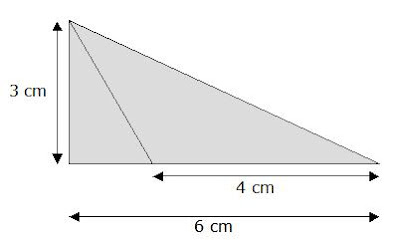The correct answer is as follows:

Area of triangle = ½ x base x height
= ½ x 4cm x 3cm = 6 square cm (correct answer)

In order to avoid making the mistake above, always remember that the base of the triangle must never extend outside the triangle.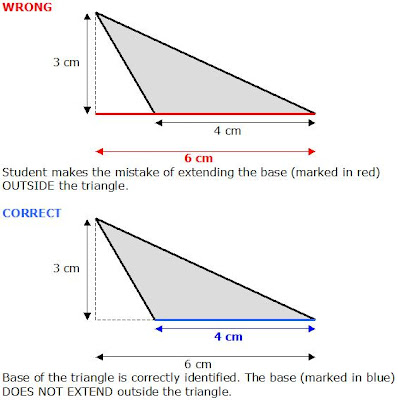## Sunday, March 01, 2009

### Nanyang Pri Sch P6 CA1 2008 Math 48

Sean and Tim had some money at first. Sean gave Tim \$60 and both of them had the same amount of money. If Tim were to return \$54 to Sean, the ratio of Sean’s money to Tim’s money would be 5:2.

a) How much money did Sean have at first?
b) What is the ratio of Sean’s money to Tim’s money at first?

Solution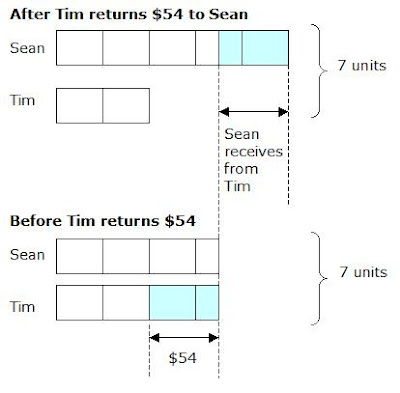1.5 units ----- \$54
1 unit ----- \$54 divided 1.5 = \$36

a)
Sean at first
3.5 units + \$60
= (3.5 x \$36) + \$60
= \$126 + \$60
= \$186

b)
After Sean gives Tim \$60, but before Tim returns \$54:-
Sean ----- \$126
Tim ----- \$126

At first,
Sean ----- \$186
Tim ----- \$126 - \$60 = \$66

Sean : Tim
186 : 66
31 : 11

Answer: The ratio was 31 : 11.

### Nanyang Pri Sch P6 CA1 2008 Math 47

The wooden box shown below has the sides 12cm by 10cm by 15cm. It has no cover at the top and has a thickness of 2cm around it.a) What is the capacity of the box?
b) What is the maximum number of 2-cm cubes that can be placed in the box?

Solution

a)
Length ----- 12cm – 2cm - 2cm = 8 cm
Breadth ----- 10cm – 2cm – 2cm = 6 cm
Height ----- 15cm – 2cm = 13 cm
Capacity ----- 8cm x 6cm x 13cm = 624 cubic cm (Answer)

b)
Length ----- 8 divided by 2 = 4
Breadth ----- 6 divided by 3 = 3
Height ----- 13 divided by 2 = 6 remainder 1

Maximum number of cubes ----- 4 x 3 x 6 = 72

Answer: A maximum of 72 cubes can be placed in the box.

### Nanyang Pri Sch P6 CA1 2008 Math 46

The figure below is made up of a rectangle, square and a shaded triangle. Find the area of the shaded triangle.SolutionArea of Triangle A ----- ½ x 1cm x 6 cm = 3 square cm
Area of Rectangle B ----- 4cm x 1cm = 4 square cm
Area of Triangle C ----- ½ x 7cm x 4cm = 14 square cm

Area of A + B + C + shaded triangle -----
½ x 8cm x 10cm = 40 square cm